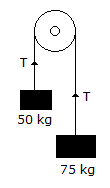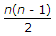# Civil Engineering - Applied Mechanics

### Exercise :: Applied Mechanics - Section 4

26.

The intrinsic equation of catenary is

 A. S = c tan ψ B. y = c cosh x/c C. y = c cosh ψ D. y = c sinh ψ.

Explanation:

No answer description available for this question. Let us discuss.

27.

A ball which is thrown upwards, returns to the ground describing a parabolic path during its flight

 A. vertical component of velocity remains constant B. horizontal component of velocity remains constant C. speed of the ball remains constant D. kinetic energy of the ball remains constant.

Explanation:

No answer description available for this question. Let us discuss.

28.

Angular acceleration of a particle may be expressed as

 A. radians/sec2 B. degrees/sec2 C. revolutions/sec D. all the above.

Explanation:

No answer description available for this question. Let us discuss.

29.

Two loads of 50 kg and 75 kg are hung at the ends of a rope passing over a smooth pulley shown in below figure. The tension in the string is :A. 50 kg B. 75 kg C. 25 kg D. 60 kg.

Explanation:

No answer description available for this question. Let us discuss.

30.

Total no of instantaneous centres of a machine having n links, is

 A. n/2 B. n C. (n - 1) D.